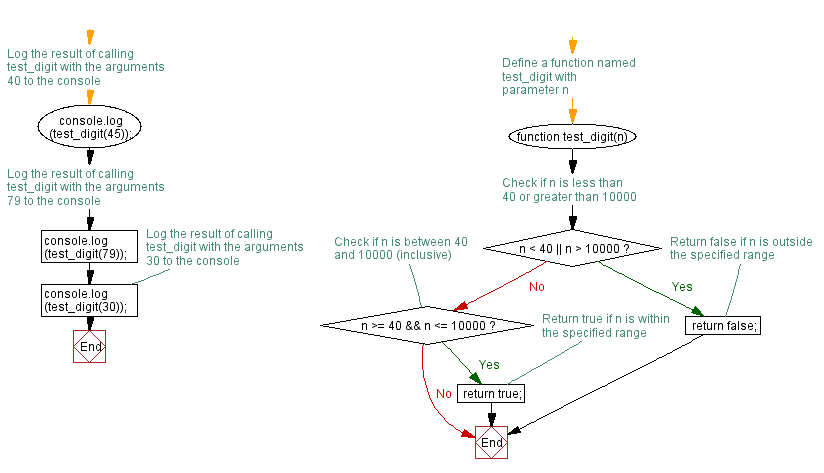# JavaScript: Check whether a given number presents in the range 40..10000

## JavaScript Basic: Exercise-47 with Solution

Write a JavaScript program to check whether a given number is presents in the range 40..10000.
For example 40 presents in 40 and 4000.

Sample Solution:

HTML Code:

``````<!DOCTYPE html>
<html>
<meta charset="utf-8">
<meta name="viewport" content="width=device-width">
<title>JavaScript program to check whether a given number is presents in the range 40..10000. </title>
<body>

</body>
</html>
```
```

JavaScript Code:

``````function test_digit(x, y, n)
{
if (n < 40 || n > 10000)
return false;
else
if (n >= x && n <= y)
return true;
else
return false;
}
console.log(test_digit(40, 4000, 45));
console.log(test_digit(80, 320, 79));
console.log(test_digit(89, 4000, 30));
```
```

Sample Output:

```true
false
false
```

Flowchart:ES6 Version:

``````function test_digit(x, y, n)
{
if (n < 40 || n > 10000)
return false;
else
if (n >= x && n <= y)
return true;
else
return false;
}
console.log(test_digit(40, 4000, 45));
console.log(test_digit(80, 320, 79));
console.log(test_digit(89, 4000, 30));

``````

Live Demo:

See the Pen JavaScript: Check whether a given number presents in the range 40..10000 - basic-ex-47 by w3resource (@w3resource) on CodePen.

Improve this sample solution and post your code through Disqus

What is the difficulty level of this exercise?

Test your Programming skills with w3resource's quiz.

﻿

## JavaScript: Tips of the Day

Checks if a string is an anagram of another string (case-insensitive, ignores spaces, punctuation and special characters)

Example:

```const isAnagram = (str1, str2) => {
const normalize = str =>
str
.toLowerCase()
.replace(/[^a-z0-9]/gi, '')
.split('')
.sort()
.join('');
return normalize(str1) === normalize(str2);
};
console.log(isAnagram('iceman', 'cinema')); // true
```

Output:

```true
```